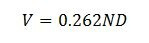INTRODUCTION:One of the major design factors in the choice of a polymer bushing is the expected operating PV.  The operating PV is the product of the surface speed of the shaft and the specific bearing load, and serves as an empirical tool for comparing the performance of different bearings.

Here are some additional articles on polymer bushings:

Polymer Bushing Equations:

The surface speed of the shaft is calculated using Equation (1) below, where V in units of in/min, N is the shaft revolutions per minute, and D is the shaft diameter in inches.  The units for surface speed are in ft/min.  Note that the surface speed is actually the speed of the wear surface.(1)

The specific bearing load (sometimes called the bearing pressure) is simply the total load divided by the projected contact area of the bushing (or bearing) surface.  This is shown in Equation (2), where Dinner is the inner diameter of the bushing and L is the length of the bushing, both in inches.(2)

For a flanged bushing, the projected area is given by Equation (3), where FL is the flange length, Ft is the thickness of the flange, all in inches.(3)

The unit pressure is then the total load F in lb divided by the projected area, shown in Equation (3) below.  The maximum unit pressure must be less than the compressive strength of the polymer.(4)

## Sample Polymer Bushing Calculation

Lets look at a sample calculation for a 1 shaft running at 250 rpm with a total load of 95 lbs and a bearing length of 0.75 in.  It also has a continuous service temperature of 200 F.When selecting a polymer for a bushing, it is necessary to check the maximum PV value, then the maximum P and maximum V for the bearing material you are considering.  In our sample calculation, our PV came out to about 8,300 psi ft/min.  If we look at a bearing material guide, such as the one here at EMC, we would see a material such as Flouron 6510 Acetal immediately stand out as a possibility, because our PV of 8,297 psi ft/min < 10,000 psi ft/min.  Our value for P is 127 psi, which is far less than its maximum P of 1,000 psi.  Our value for V is about 66 ft/min, which is less that the materials maximum V of 66 ft/min.

However, our material choice is not complete!  We also need to check the continuous running temperature.  Flouron 6510 Acetal has a continuous service temperature of 180 F.  This becomes a problem, because the expected continuous service temperature for our bearing is 200 F.  That means we need to keep looking for a material.

CONCLUSION:

Again, when selecting an appropriate polymer bushing for your application, one of the most important factors is the PV, which is based on the surface speed of the shaft and the expected bearing load.  This should be your first step in designing the bushings.